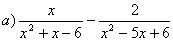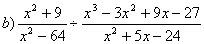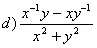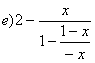KING FAHD UNIVERSITY OF PETROLUEM AND MINERALS

Faculty of Science – Math Prep-Year Program

Math 001 - Term 031

Worksheet (P5)

Q1) Factor each of the following completely :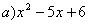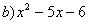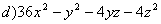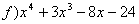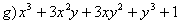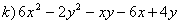Q2) Find all positive values of k such that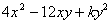is a perfect-square

trinomial.

KING FAHD UNIVERSITY OF PETROLUEM AND MINERALS

Faculty of Science – Math Prep-Year Program

Math 001 - Term 022

Worksheet (P6)

Simplify :